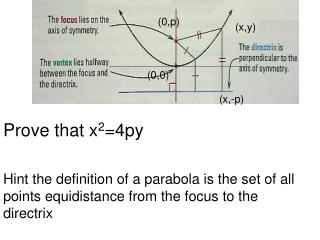DownloadDownload PresentationProve that x 2 =4py Hint the definition of a parabola is the set of all points equidistance from the focus to the direct

# Prove that x 2 =4py Hint the definition of a parabola is the set of all points equidistance from the focus to the direct

Download Presentation## Prove that x 2 =4py Hint the definition of a parabola is the set of all points equidistance from the focus to the direct

- - - - - - - - - - - - - - - - - - - - - - - - - - - E N D - - - - - - - - - - - - - - - - - - - - - - - - - - -
##### Presentation Transcript

1. (0,p) (x,y) (0,0) (x,-p) Prove that x2=4py Hint the definition of a parabola is the set of all points equidistance from the focus to the directrix

2. Conic sections are the sections that can be formed by intersecting a plane and a double-napped cone, as shown below.

3. The beam of light from a flashlight is a cone. When the light hits a flat surface such as a wall, the edge of the beam of light forms a conic section.

4. 10.3 Circles Purpose Graph and write equations of circles.

5. Definition of a circle Set of all points equidistant from a fixed point. What is the equation of a circle. (x,y) (0,0)

6. Standard form of a circle x2+y2 = r2

7. Graph the circle and give the radius x2+y2 = 36

8. Is the a parabola or a circle?

9. Write the equation of the circle with the given radius and whose center is the origin. Radius =3 Radius = Radius =2

10. Write the equation of the circle with the given point on the circle. (5,12) (8,15)

11. A tangent to a circle is perpendicular to the radius drawn to the point on the circle

12. Write an equation of the line that is tangent to the circle x2+y2 = 13at (2,3)

13. Representing regions inside and outside the circle x2+y2 = r2 Inside would be what symbol? Outside would be that symbol?

14. The beam of a lighthouse can be seen for up to 20 miles. You are on a ship that is 10 miles north and 16 miles north of the lighthouse .A. Write and inequality to describe the region lit by the lighthouse beam.B. Can you see with the light house beam?

15. Day 1 P.604-606 # 1-4,7-19(by 3);20-75(by 5),88,89

16. Review 10.1-10.3P. 607 # 1-23(non mult. of 3)